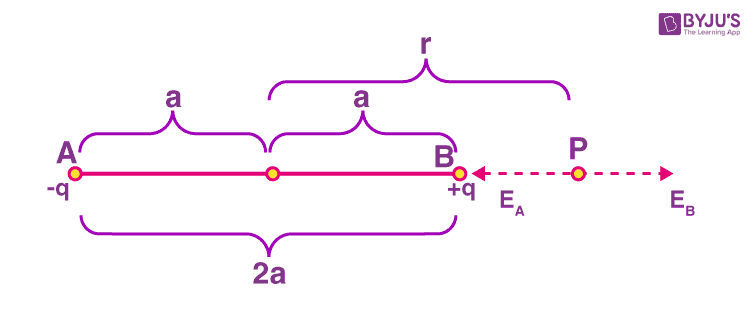# Electric Field Intensity at any point due to an Ideal Dipole

## What is an Electric Field Intensity?

The space around an electric charge in which its influence can be felt is known as the electric field. At a point, the electric field intensity is the force experienced by a unit positive charge placed at that point. The electric field intensity due to a positive charge is always directed away from the charge and the intensity due to a negative charge is always directed towards the charge. Electric Field Intensity is a vector quantity.

## What is an Electric Dipole?

An electric dipole is defined as a pair of opposite charges q and –q separated by a distance d.

## Electric Field Intensity of an Electric Dipole Derivation

### Derivation of Electric Field Intensity for points on the Axial Line of a DipoleConsider a system of charges -q and +q separated by a distance 2a. Let “P” be any point on the axial line where the electric field intensity needs to be determined.

Electric Field at P (EB) due to +q is given as follows:

$$\begin{array}{l}E_{B}=\frac{1}{4\pi \varepsilon _{0}}\frac{q}{BP^2}\end{array}$$

$$\begin{array}{l}E_{B}=\frac{1}{4\pi \varepsilon _{0}}\frac{q}{(r-a)^2}\end{array}$$

The electric field at P (EA) due to -q is given as follows:

$$\begin{array}{l}E_{A}=\frac{1}{4\pi \varepsilon _{0}}\frac{q}{(AP)^2}\end{array}$$

$$\begin{array}{l}E_{A}=\frac{1}{4\pi \varepsilon _{0}}\frac{q}{(r+a)^2}\end{array}$$

The following equation gives the net field at point P:

$$\begin{array}{l}E_{P}=E_{B}-E_{A}\end{array}$$

$$\begin{array}{l}=\frac{1}{4\pi \epsilon _{0}}\frac{q}{(r-a)^2}-\frac{q}{(r+a)^2}\end{array}$$

Simplifying the above equation, we get

$$\begin{array}{l}E_{p}=\frac{q}{4\pi \epsilon _{0}}\times \frac{2r}{(r^2-a^2)^2}\end{array}$$

Further simplifying, we get

$$\begin{array}{l}E_{p}=\frac{2kpr}{(r^2-a^2)^2}\end{array}$$

In the equation, p = 2aq and

$$\begin{array}{l}k = \frac{1}{4\pi \epsilon _{0}}\end{array}$$